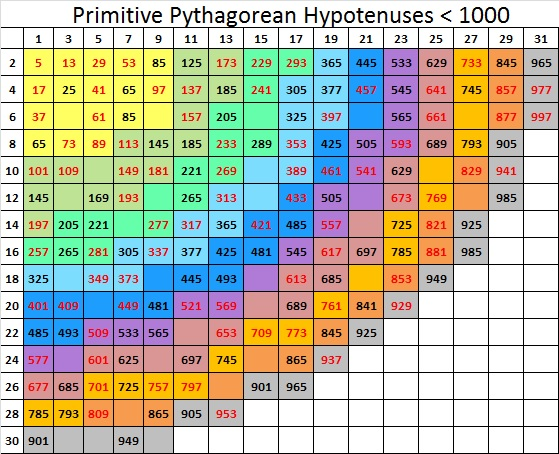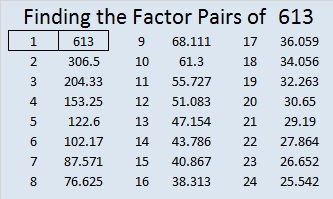# I can tell that 613 is a prime number just by looking at it!

613 is the 18th centered square number because 17 and 18 are consecutive numbers and 18² + 17² = 613.613 is the hypotenuse of the primitive Pythagorean triple 35-612-613, which was calculated using (18^2) – (17^2), 2 x 17 x 18, and (18^2) + (17^2).

Knowing that (18^2) + (17^2) = 613, I can tell that 613 is a prime number just by looking at it! Here’s what I see:

• 613 obviously is not divisible by 5.
• It is almost as obvious that 613 is not divisible by 13.
• 18^2 is not divisible by 17 so (18^2) + (17^2) is not divisible by 17. Thus 613 is not divisible by 17.
• Since 613 is not divisible by 5, 13, or 17, it is a prime number.

“What?” you say. What about all the other possible prime factors less than 24.8, the approximate value of √613? Don’t we need to TRY to divide 613 by 2, 3, 7, 11, 19, and 23?

No, we don’t. Those numbers don’t matter at all. Since 17^2 + 18^2 = 613, and because 17 and 18 have NO common prime factors and only one of them is odd, I already know that none of those numbers will divide into 613. Let me show you what I mean.

Look at this chart of ALL the primitive Pythagorean triple hypotenuses less than 1000:The numbers in red are all prime numbers and appear on the chart only one time. Every prime number less than 1000 that can be written in the form 4N + 1 appears somewhere on the chart. 613 can be found at the intersection of the column under 17 and the row labeled 18.

The black numbers on the colorful squares are composite numbers and many of them appear on the chart above more than one time. There is something very interesting about the prime factors of these composite numbers. Every single one of those factors is also the hypotenuse of a primitive Pythagorean triple! Also notice that at least one of those factors is less than or equal to the square root of the composite number. Here is a chart of those composite numbers, how many times they appear on the chart above, and their prime factorizations:Also notice that if you divide any of the above composite numbers OR their factors by 4, the remainder is always 1.

I have demonstrated that the following is true for any integer less than 1000. I wasn’t sure if it had been proven for integers in general so I asked Gordan Savin, a professor at the University of Utah, who teaches number theory. He informed me that it has been proven and that it follows from the uniqueness of factorization of Gaussian integers.

In conclusion, if an integer can be written as the sum of two squares, one odd and one even, and the numbers being squared have no common prime factors, then ALL the factors of that odd integer will be hypotenuses of primitive Pythagorean triples. If the integer is a composite number, at least one of those primitive hypotenuses will be less than or equal to the square root of that integer.

Here’s the same thing in more standard mathematical language:

If a natural number of the form 4N + 1 can be written as the sum of two squares (a^2)+ (b^2) and if a and b have no common prime factors, then ALL the factors of 4N + 1 will be of the form 4n + 1. If 4N + 1 is a composite number, there will exist at least one prime factor, 4n + 1 ≤  √(4N + 1). If no such factor exists, then 4N + 1 is a prime number.

• 613 is a prime number.
• Prime factorization: 613 is prime.
• The exponent of prime number 613 is 1. Adding 1 to that exponent we get (1 + 1) = 2. Therefore 613 has exactly 2 factors.
• Factors of 613: 1, 613
• Factor pairs: 613 = 1 x 613
• 613 has no square factors that allow its square root to be simplified. √613 ≈ 24.7588How do we know that 613 is a prime number? If 613 were not a prime number, then it would be divisible by at least one prime number less than or equal to √613 ≈ 24.8. Since 613 cannot be divided evenly by 2, 3, 5, 7, 11, 13, 17, 19, or 23, we know that 613 is a prime number. But as I explained above since (18^2) + (17^2) = 613, and consecutive integers 18 and 17 have no common prime factors, it is only necessary to check to see if 613 is divisible by 5, 13, or 17. Since it isn’t divisible by any of those three numbers, 613 is a prime number.

## 4 thoughts on “I can tell that 613 is a prime number just by looking at it!”

1.Paula Beardell Krieg

Great chart and graphics!!! I will be printing out this post and going through each piece of it slowly until I’m fully comfortable with all the different ways that you are unpacking this number. 🙂

•ivasallay

Thank you! I hope I was able to write clearly enough that you will get fully comfortable with it.

You were part of my inspiration while I wrote this post because you once commented, “439 just has that prime look to it.” What I wrote in this post does not apply to the number 439 because 439 ÷ 4 has a remainder of 3, but I still thought of you when I came up with the title and while I wrote the post.

2.spinbbtgmailcom

Thank you. I was looking for pomegranates seeds number theories. Here is the best math description of it.

•ivasallay

Thanks for your comment. It helped me learn something new. I did not know about 613 seeds in pomegranates or 613 commandments in the Torah. Thanks again.

This site uses Akismet to reduce spam. Learn how your comment data is processed.